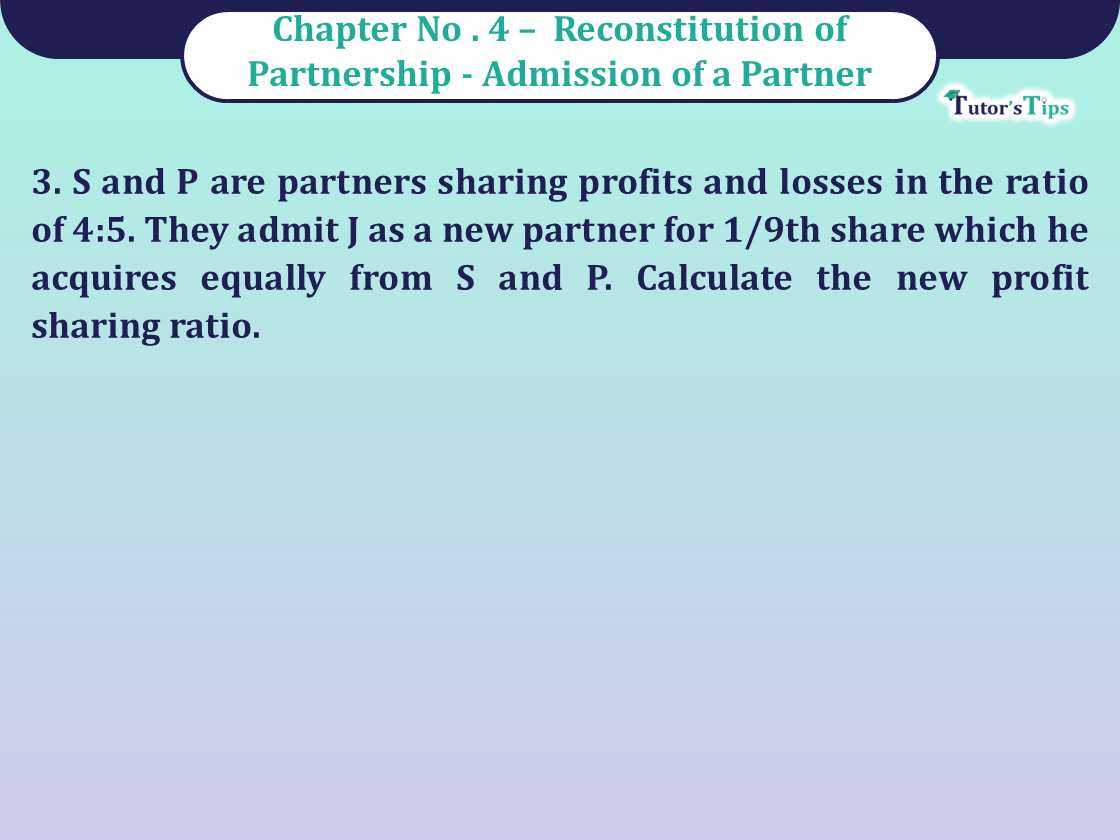# Question 3 Chapter 4 of Class 12 Part – 1 VK PublicationQuestion 3 Chapter 4 of Class 12 Part – 1

3. S and P are partners sharing profits and losses in the ratio of 4:5. They admit J as a new partner for 1/9th share which he acquires equally from S and P. Calculate the new profit sharing ratio.

## The solution of Question 3 Chapter 4 of Class 12 Part – 1: –

Old Ratio of S and P = 4:5
J is admitted for 1/9th share

 J acquires = 1 x 1 9 2

 = 1 from S 18
 S’s New Share = 4 – 1 9 18
 = 7 18
 J acquires = 1 x 1 9 2
 = 1 18
 P’s New share = 5 – 1 9 18

 = 9 18
 J’s New Share = 1 or 1 9 18

New profit-sharing ratio of S, P, J = 7:9:2

Comment if you have any questions.

Also, Check out the solved question of previous Chapters: –